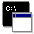## Firefly and PC GAMESS-related discussion club

Learn how to ask questions correctly'Abnormal Energy' obtained in MP4 single point energy calculation
Hello everyone!

I am currently running an MP4 single point energy calculation (basis set: 6-311++G**) on a few hydrocarbons using Firefly version 7.1.G in one of the PCs in our laboratory. The geometry I used for the single point energy calculation was the equilibrium geometry I obtained from my MP2 optimization calculation using the same molecule. On some of my molecules examined at MP4, I found out that the energy calculated was 'abnormally' large(i.e. the absolute value of the energy calculated) that my succeeding calculations (i.e. enthalpy of reaction, enthalpy of activation, etc. ) doesn't make any sense at all. I checked my input files, they were all the same. I just changed the geometry. Shown below is the fraction of my result wherein the energy I label as 'abnormal' is reflected.

RESULTS OF MOLLER-PLESSET 4TH ORDER CORRECTION ARE

а а а а а аE(RHF) а а а = а а а-309.9452940908

а а а а а аE(D,2) а а а = а а а а-1.1646736559
а а а а а аE(MP2) а а а = а а а-311.1099677467

а а а а а аE(D,3) а а а = а а а а-0.0698223432
а а а а а аE(D,2+3) а а = а а а а-1.2344959991
а а а а а аE(MP3) а а а = а а а-311.1797900899

а а а а а аE(S,4) а а а = а а а а-0.0443751557
а а а а а аE(D,4) а а а = а а а-105.3701897285
а а а а а аE(D,2+3+4) а = а а а-106.6046857276
а а а а а аE(T,4) а а а = а а а а-0.0706244480
а а а а а аE(R+Q,4) а а = а а а а 0.0297425817
а а а а а аE(SDTQ,4) а а= а а а-105.4554467506
а а а а а аE(SDTQ,2+3+4)= а а а-106.6899427497
а а а а а аE(MP4-SDTQ) а= а а а-416.6352368404

..... DONE WITH MP4 ENERGY .....

аI also attached the output file of this calculation. What might be the problem in this calculation? Can I ask for suggestions on how to improve/solve such? Any help is highly appreciated. Thank you in advance!

Regards,
XaizaThis message contains the 1114 kb attachment [ single_point.log ] output file containing 'abnormal energy'

[ This message was edited on Wed Jun 26 '13 at 2:49pm by the author ]Wed Jun 26 '13 2:49pmThis message read 1185 times